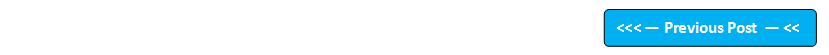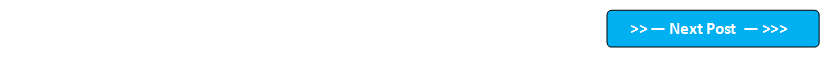## Blog Pages

### 185. varargs 'Variable length arguments'varargs (variable length arguments) is a unique concept in Java.

Reason behind the origin of varargs -
1. What will happen if you don't know how many arguments you need to be passed to a method ?
2. What will happen if you want to pass unlimited variables to a method ?
In order to answer the above two questions, Java has introduced a unique concept called varargs.

Using varargs you can pass as many as arguments of the same type to any method. So varargs allows the method to accept zero to unlimited number of arguments.

Syntax of varargs -

varargs (variable length argument) is specified by three periods (...)
In Syntax, we need to specify these three periods (...) after the data type as shown below -

return_type method_name(data_type ... variable_name)
{
//Method code
}

Example of varargs -

void sum(int... var)
{
//Method code
}

Lets implement varargs on Eclipse IDE -

1. Launch Eclipse IDE, create a Java Project 'Project 019' as shown below -

2. Create a Class 'VarargsDemo' as shown below -

3. Create a method sum( ) by specifying integer type varargs argument as shown below -

4. Observe that an error is displayed on the above step, click on the error and view the error message as shown below -

This error is displayed even though I have installed Java version 1.8 in my system which is greater than 1.5.

Why is this error displayed and how to resolve this error ?

Though we have installed Java version 1.8 in our system, the Eclipse IDE has set the Compiler compliance level which is less than 1.5.

How to resolve this error ?

We can resolve this error by selecting the 'Change project compliance and JRE to 1.5' option in the above displayed error dialog or just follow the below steps to change the compiler compliance level from a differenr place in Eclipse IDE.

5. Right click on the Java Project 'Project 019' and select the 'Properties' option

6. In 'Properties for Project 019' dialog, select 'Java Compiler' on the left and observe that 'Compiler Compliance Level' option is displayed on the right as shown below -

7. Observe that the Compiler Compliance Level is set to 1.4 (i.e. less than 1.5) by default in my machine as shown below -

8. Change the compliance level to 1.5 and click on 'Apply' followed by 'OK' buttons as shown below -

9. Click on 'Yes' button to rebuild the project if any dialog asking to rebuild is displayed

10. Observe that the error got resolved in the VarargsDemo Class as shown below -

11. Now write the code to calculate the sum of all the integer arguments passed to the varargs argument as shown below -

12. Print the calculated sum as shown below -

13. Now create main( ) method in the same Class to call the sum( ) method by passing different number of parameters to the same method as shown below -

14. Observe that errors are displayed in the above screen. This is because, as the main( ) is a static method, it can only access the methods that are static only. Hence it is throwing these errors. In order to resolve make the sum( ) method as static as shown below and observe that the errors got resolved -

15.  Run the Class and observe that the sum( ) method has accepted different number of arguments passed to it using varargs arguments and the sum of the passed arguments is calculated and printed in as output in the console as shown below -